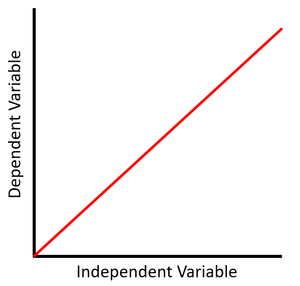# Directly Proportional

## Key Stage 4

### Meaning

When two variables are directly proportional when one variable is multiplied by a factor, the other variable is multiplied by the same factor.

A scatter graph showing a directly proportional relationship has line with a linear gradient that passes through zero (it has a y-intercept of zero).
On a proportional scatter graph when one variable doubles, the other doubles or when one triples the other triples.
When two variables are directly proportional when any value for y is divided by its corresponding value for x it will always give a constant value.
Two variables are said to be directly proportional when they always vary by the same ratio.

### Exam Marks

Straight line [1 mark]
Passing through zero / passing through the origin [1 mark]

### ExamplesThis scatter graph shows a linear relationship that is directly proportional where x doubles, y doubles. \(y = mx\) Where m, the gradient, is positive.